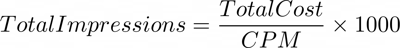Features

# Cost Per Thousand Calculator

Cost per thousand (CPM) is a marketing metric used to indicate the cost per thousand impressions.

Short for cost per mille and more often referred to as cost per thousand, CPM is a core metric used in marketing and media buying. CPM represents the average cost

## CPM Formula

### Calculate CPM

Cost Per Thousand Impressions (CPM) is calculated by dividing the total cost by the total impressions divided by 1000.### Calculate Cost

Starting with the CPM and impressions the total cost can be calculated by multiplying the CPM by the total number of impressions divided by 1000.### Calculate Impressions

To calculate how many impressions will be purchased based on the CPM and total cost. Start by divining the total cost by the CPM and then multiply this answer by 1000. Remember when looking at the final answer it is not possible to have partial ad impressions, so if the number contains decimal places you will need to round down to the nearest whole number.